# Spacecraft telemetry and command¶

#### V. Hunter Adams (vha3), MAE 4160/5160, Spring 2020¶

In :
from IPython.display import Latex
from IPython.display import Image
from IPython.core.display import HTML


## In these lectures:¶

2. Modulations
3. Bit error rate
4. The Shannon Limit
5. Coding Techniques
6. Antennas

The goal of a link budget is to determine the signal-to-noise ratio of a transmitted signal. In the case of spacecraft, these are often radio signals, though they could also be optical signals. I want to construct this lecture and the next lecture by starting with an intuitive skeleton of the link budget equation, and then stepping through each term to fill in its associated details. Once we've arrived at the link budget equation, we'll use it to perform some other common analyses, like calculating bit error rate and channel capacity.

The most general expression for signal-to-noise ratio is, by definition, the received power of the signal divided by the power of the noise:

\begin{align} SNR &= \frac{P_R}{P_N} \end{align}

Depending on the units in which $P_R$ and $P_N$ are represented, you may see the variable $SNR$ given different neames. $\frac{E_b}{N_0}$ is a common one. We'll discuss all of these minor details throughout the coming two lectures, but the above expression summarizes our goal most generally. When link budgeting, we decide on some threshold signal-to-noise ratio which is acceptable, and then we design our telemetry and command system such that we achieve that threshold $SNR$.

### Free-space transmission¶

Consider the numerator of the $SNR$ equation $SNR = \frac{P_R}{P_N}$. This represents the power of the received signal. That is to say, the power of the signal after it has been transmitted through an antenna, through space, and into another antenna. For the time being, we are assuming that there is no noise in the system. We are allowed to do this because all of those noise sources appear in the denominator, so we'll consider them separately.

Question: Before we even get started computing this quantity, what parameters do you expect will appear in the equation for the received power? An example of one such parameter is the distance of the transmission. What else will appear?

1. Transmit power.
2. Transmission distance.
3. TX antenna gain.
4. RX antenna gain.
5. Wavelength of carrier wave.
6. (Coming later) Coding gain.

Let's consider this systematically. Let us consider the case of two antennas (transmit and receive antennas) in free space separated by a distance $R$.

Let us assume that $P_T$ Watts of total power is delivered to the transmit antenna, which (for the moment) is assumed to be omnidirectional and lossless. Furthermore, we'll assume that the receive antenna is in the far field of the the transmit antenna (a safe assumption for transmissions from orbit). As the signal propagates spherically out from the transmit antenna, the power density (watts per square meter) of the plane wave decreases. By the time the signal reaches the receive antenna at a distance $d$ away, the power density is given by:

\begin{align} p &= \frac{P_T}{4\pi d^2} \end{align}
In :
Image("freespace.png", width=400)

Out: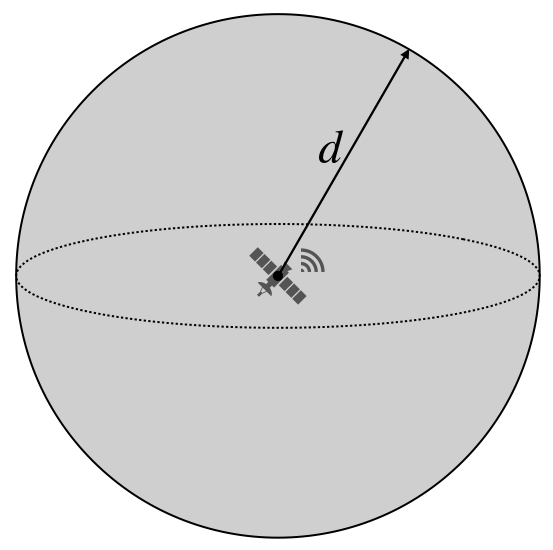Any losses and directionality of the transmit antenna can be absorbed by a gain $G_T$. A transmit gain greater than one for a lossless antenna means that it is transmitting in a preferrred direction, and that direction is towards the receive antenna. A gain of 1 corresponds to an isotropic antenna. Augmenting the above equation:

\begin{align} p &= \frac{P_T}{4\pi d^2} G_T \end{align}

Now consider the receive antenna. The aperature (i.e. effective area or receiving cross section) of an antenna is a measure of how effective an antenna is at receiving the power of radio waves. It is the area, oriented perpendicular to the direction of an incoming radio wave, that would intercept the same amount of power from that wave as is produced by the antenna receiving it. We can therefore augment the equation again to get received power:

\begin{align} P_R &= \frac{P_T}{4\pi d^2}G_T A_{ER} \end{align}

The aperature for any antenna can also be expressed as:

\begin{align} A_{ER} &= \frac{\lambda ^2}{4\pi}G_R \end{align}

Rewriting again:

\begin{align} P_R = \frac{P_TG_TG_R\lambda^2}{\left(4\pi d\right)^2} \end{align}

The above expression has a name. It is the Friis Transmission Formula. This expression gives us the received power as a function of transmitted power, tx antenna gain, rx antenna gain, tx distance, and wavelength. In the above equation, power is measured in linear units (watts). If, however, we convert to logarithmic units (decibels), we get the following:

\begin{align} 10\text{log}_{10}P_R = 10\text{log}_{10}\left(\frac{P_TG_TG_R\lambda^2}{\left(4\pi d\right)^2}\right) \end{align}
\begin{align} [P_R]_{db} = [P_T]_{db} + [G_T]_{db} + [G_R]_{dB} + 10\text{log}_{10}\left[\left(\frac{\lambda}{4\pi R}\right)^{2}\right] \end{align}

### Attenuation¶

Of course, the channel through which we are communicating is not lossless. There are various sources of attenuation which decrease the strength of the signal. The attenuation comes from the atmosphere and from losses within the transmit and receive hardware itself. Our expression for the losses will include:

1. Atmospheric losses
2. Circuit losses

#### The atmosphere¶

Transmission through the atmosphere attenuates the signal by some scale factor $L_a$. This will be some number between 0 and 1. We can augment our equation above for the received power to include attenuation from the atmosphere as shown below:

\begin{align} P_R = \frac{P_TG_TG_RL_a\lambda^2}{\left(4\pi d\right)^2} \end{align}

The amount of attentuation caused by the atmosphere depends on one's choice of frequency, as shown below.

In :
Image("att.png", width=800)

Out: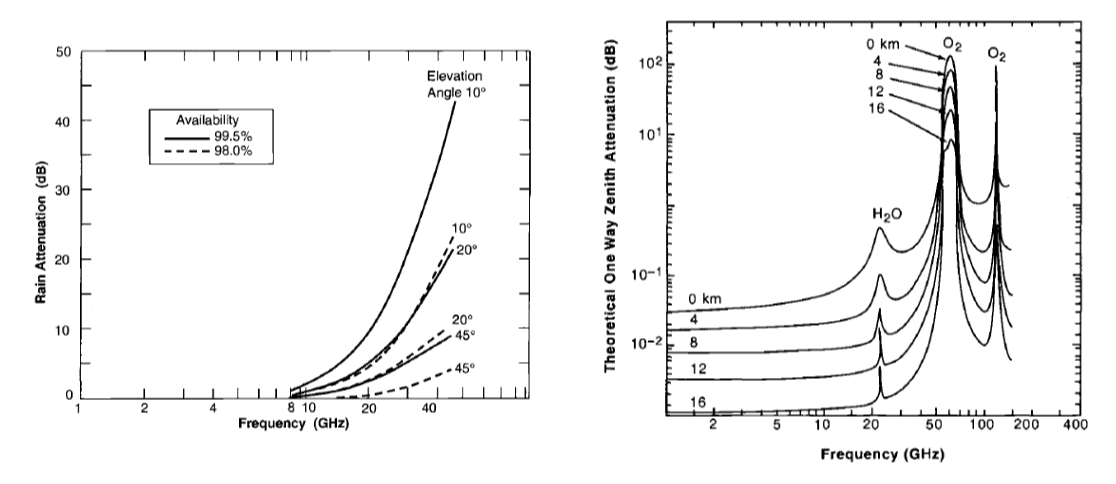#### Circuit losses¶

Our signal will also be attenuated by our hardware (coaxial cables, connectors, etc.). Transmission through this hardware will attenuate the signal by some scale factor $L_l$. So, we can again augment our equation for the received power:

\begin{align} P_R = \frac{P_TG_TG_RL_aL_l\lambda^2}{\left(4\pi d\right)^2} \text{ (Watts)} \end{align}

### Changing units¶

The above expression gives us the received power (energy per unit time, i.e. J/s, i.e. Watts) at the Rx antenna, and includes information about the tx power, tx gain, rx gain, atmospheric attenuation, circuit attenuation, tx distance, and wavelength. For doing a link budget analysis, we would like to know the energy per unit bit. We want to know how much energy is contained within each bit, which we represent using the variable $E_b$ (for Energy per bit). To find $E_b$, we simply divide the received power by the bit rate (bits/sec):

\begin{align} E_b &= \frac{P_R}{R_b}\\ &= \frac{P_TG_TG_RL_aL_l\lambda^2}{\left(4\pi d\right)^2R_b} \text{ (Joules/bit)} \end{align}

### Noise¶

We want to compare the energy contribution from our signal (above) to the energy contribution from noise. We find the contribution from noise by calculating the noise spectral density, which is the noise power per unit of bandwidth. In our case, the majority of our noise contribution comes from thermal noise. The spectral noise density for thermal noise is calculated as shown below:

\begin{align} N_0 &= \frac{P_N}{B} = K_B T_{sys} \end{align}

Where $K_B$ is the Boltzmann Constant (units of Joules/Kelvin), and $T_{sys}$ is the system noise temperature (Kelvin). $P_N$ is the noise power (Watts) and $B$ is the bandwidth (Hz, bits/sec).

#### System noise temperature¶

The total input noise temperature on the system, $T_{sys}$, has contributions from the antenna and the receiver:

\begin{align} T_{sys} &= T_A + T_{R} \end{align}

The antenna noise temperature $T_A$ gives the noise power seen at the output of the antenna. The noise temperature of the receiver circuitry $T_R$ represents noise generated by noisy components inside the receiver. The noise introduced by the antenna is thermal in nature, and depends on the frequency and on where the antenna is pointed (cold space or hot Earth?).

The receiver noise temperature $T_R$ is usually represented in terms of a noise factor $F$. The noise factor specifies the increase in noise power (referred to the input of an amplifier) due to a component or system when its input noise temperature is $T_0 = 290K$.

\begin{align} T_{R} &= T_0(F-1)\text{ , $T_0=290k$} \end{align}

where

\begin{align} F &= \frac{T_0 + T_R}{T_0} \end{align}

We often see the noise factor expressed in decibels, in which case it is called the noise figure:

\begin{align} F_N &= 10 \log_{10}\left(F\right) \end{align}

From Wikipedia: The noise figure can also be seen as the decrease in signal-to-noise ratio (SNR) caused by passing a signal through a system if the original signal had a noise temperature of 290 K. This is a common way of expressing the noise contributed by a radio frequency amplifier regardless of the amplifier's gain. For instance, assume an amplifier has a noise temperature 870 K and thus a noise figure of 6 dB. If that amplifier is used to amplify a source having a noise temperature of about room temperature (290 K), as many sources do, then the insertion of that amplifier would reduce the SNR of a signal by 6 dB. This simple relationship is frequently applicable where the source's noise is of thermal origin since a passive transducer will often have a noise temperature similar to 290 K.

Putting things back together:

\begin{align} T_{sys} &= T_A + T_0\left(F-1\right) \end{align}

Some example values below:

In :
Image("vals.png", width=800)

Out: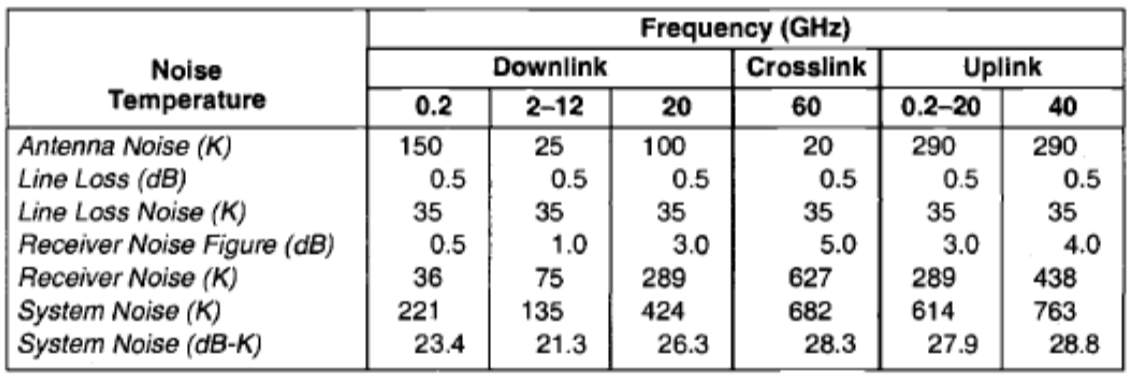We are interested in the ratio of signal energy to noise energy for each bit. We now have everything that we need in order to compute that. The signal energy per bit is given by $E_b$, and the noise energy is given by $N_0$. To get the ratio, we simply divide:

\begin{align} \boxed{\frac{E_b}{N_0} = \frac{P_TG_TG_RL_aL_l\lambda^2}{\left(4\pi d\right)^2K_BT_{sys}R_b} } \longrightarrow \text{ signal to noise ratio per bit} \end{align}

There are a couple more versions of this equation that it will be useful to have. Suppose that instead of the signal to noise ratio per bit, we wanted the signal to noise ratio over some bandwidth $B$ (measured in Hz). We can get that expression directly from the one above, by simply multiplying by $\frac{R_b}{B}$:

\begin{align} \boxed{\frac{S}{N}= \frac{P_TG_TG_RL_aL_l\lambda^2}{\left(4\pi d\right)^2K_BT_{sys}B} } \longrightarrow \text{ signal to noise ratio per bandwidth} \end{align}

It is often useful to represent the above equations in decibels.

\begin{align} \boxed{\left[\frac{S}{N}\right]_{db} = [P_T]_{db} + [G_T]_{db} + [G_R]_{dB} + [L_a]_{dB} + [L_l]_{dB} + 10\text{log}_{10}\left[\left(\frac{\lambda}{4\pi R}\right)^{2}\right] - 10\log_{10}\left(K_BT_{sys}B\right)} \end{align}
\begin{align} \boxed{\left[\frac{E_b}{N_0}\right]_{db} = [P_T]_{db} + [G_T]_{db} + [G_R]_{dB} + [L_a]_{dB} + [L_l]_{dB} + 10\text{log}_{10}\left[\left(\frac{\lambda}{4\pi R}\right)^{2}\right] - 10\log_{10}\left(K_BT_{sys}R_b\right)} \end{align}

And finally, we often see the $\frac{E_b}{N_0}$ expression defined in decibel form, using the particular set of variables defined below:

\begin{align} P_TG_T&: \text{ Equivalent Isotropic Radiated Power (EIRP)}\\ \left(\frac{\lambda}{4\pi d}\right)^2 &: \text{ Free Space Loss ($L_s$)}\\ \frac{G_R}{T_{sys}}&: \text{ Receiver gain to noise temperature} \end{align}

Rewriting the above expression in terms of these new variables:

\begin{align} \frac{E_b}{N_0} = EIRP \cdot \frac{L_aL_lL_s}{K_BR_b} \cdot \frac{G_R}{T_{sys}} \end{align}

In logarithmic form:

\begin{align} \boxed{\left[\frac{E_b}{N_0}\right]_{dB} = \left[EIRP\right]_{dB} + \left[L_a\right]_{dB} + \left[L_l\right]_{dB} + \left[L_s\right]_{dB} + \left[\frac{G_R}{T_{sys}}\right]_{dB} - \left[K_B\right]_{dB} - \left[R_b\right]_{dB} } \end{align}

## Modulations¶

In order to communicate information between satellite and ground station, we change some property of a high-frequency carrier signal $c(t)$ (amplitude, frequency, or phase) in a way that encodes the information in our message $m(t)$. This process is called modulation. Modulation is required because, if we were to transmit our information $m(t)$ directly, it would be at very low frequency (compared to the frequency of the carrier signals). These low frequency transmissions would require long antennas, and would be reflected off the ionosphere.

There are a variety of flavors of modulation.

### Analog Modulations¶

In analog modulation, the modulation is applied continuously in response to the analog information signal.

#### Amplitude modulation¶

As the name suggests, amplitude modulation encodes information in the amplitude of the carrier wave. A high-frequency signal is mixed with (multiplied by) a second, low-frequency wave that encodes the information.

\begin{align} \text{Carrier}&: c(t) = A_c\cos{\left(\omega_c t\right)}\\ \text{Signal}&: m(t) \text{ with bandwidth $B$, typically, $B<<\omega_c$}\\ \text{AM Signal}&: s_{AM} = A_cm'(t) \cos{(\omega_c t)} \text{, where $m't(t) = 1+m(t)$} \end{align}
In :
Image("am.png", width=400)

Out: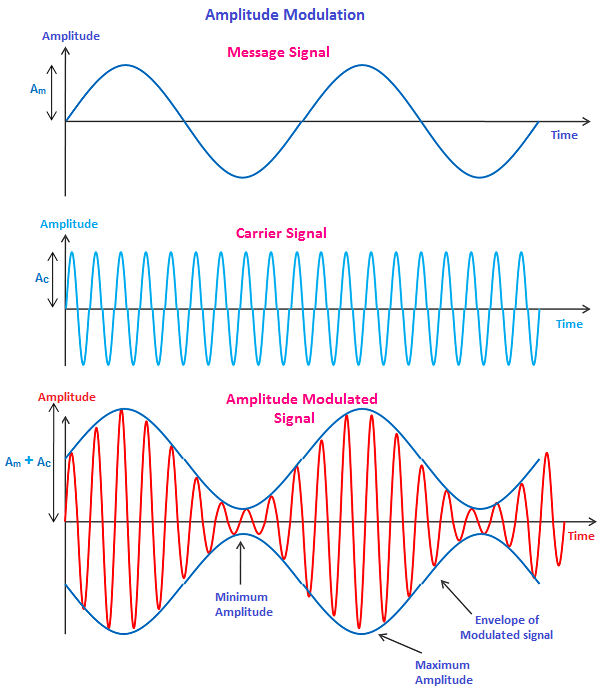AM modulation requires a local oscillator to generate the high-frequency carrier, a mixer to multiply the two signals, and an amplifier. On the receiver side, demodulation requires another local oscillator to generate a proxy of the carrier signal, a mixer to multiply, a low-pass filter to keep only the low-frequency part of the received signal, and a diode to remove the DC offset.

#### Frequency modulation¶

In analog frequency modulation, such as FM radio broadcasting of an audio signal representing voice or music, the instantaneous frequency deviation, the difference between the frequency of the carrier and its center frequency, is proportional to the modulating signal.### Digital modulations¶

In digital modulation, we use a finite number of analog signals (pulses) to represent pieces of a digital message.

#### Amplitude shift keying¶

With binary amplitude shift keying (2-ASK), we encode a 0 as $A_0 \cos{\omega_ct}$ and a 1 as $A_1\cos{\omega_ct}$. However, we could have 4-ASK, 8-ASK, etc.

In :
Image("ask.jpg", width=400)

Out: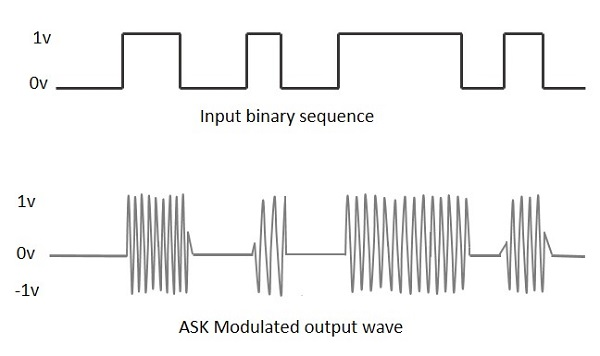#### Frequency shift keying¶

In FSK, different signals are transmitted as different frequencies. As with ASK, we can have 2FSK, 4FSK, etc. depending how many different frequencies we use to encode information.

In :
Image("fsk.png", width=400)

Out: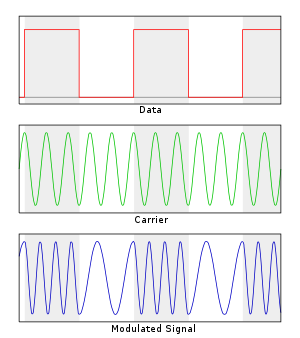#### Phase shift keying¶

In PSK, symbols correspond to different phases, as shown below for binary phase shift keying (BPSK), quadrature phase shift keying (QPSK) and 8-PSK.

In :
Image("psk.png", width=400)

Out: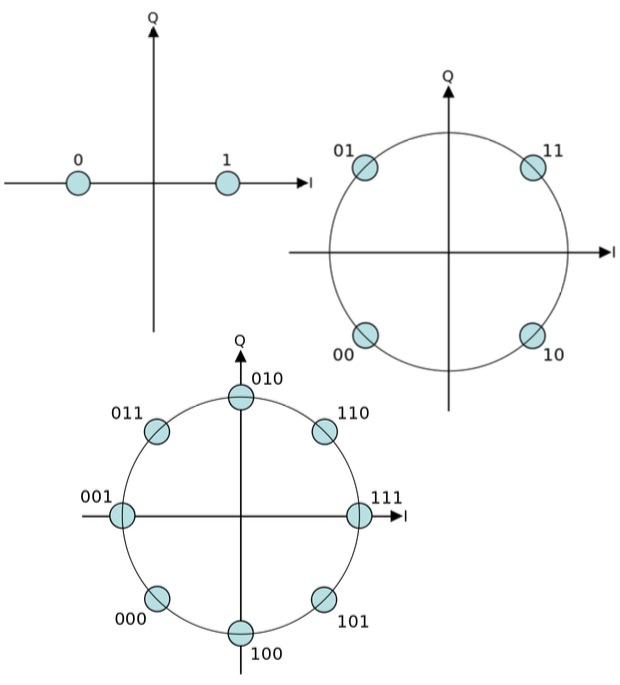### An aside on demodulation for the Monarch chip-satellites¶

The Monarch chipsats use a radio transciever that encodes information using GFSK. The receiver is an RTL-SDR software-defined radio which outputs raw I/Q data. This raw I/Q data is then demodulated into the bitstring.

In :
Image(filename = "Monarch.jpg", width=500, height=800)

Out: